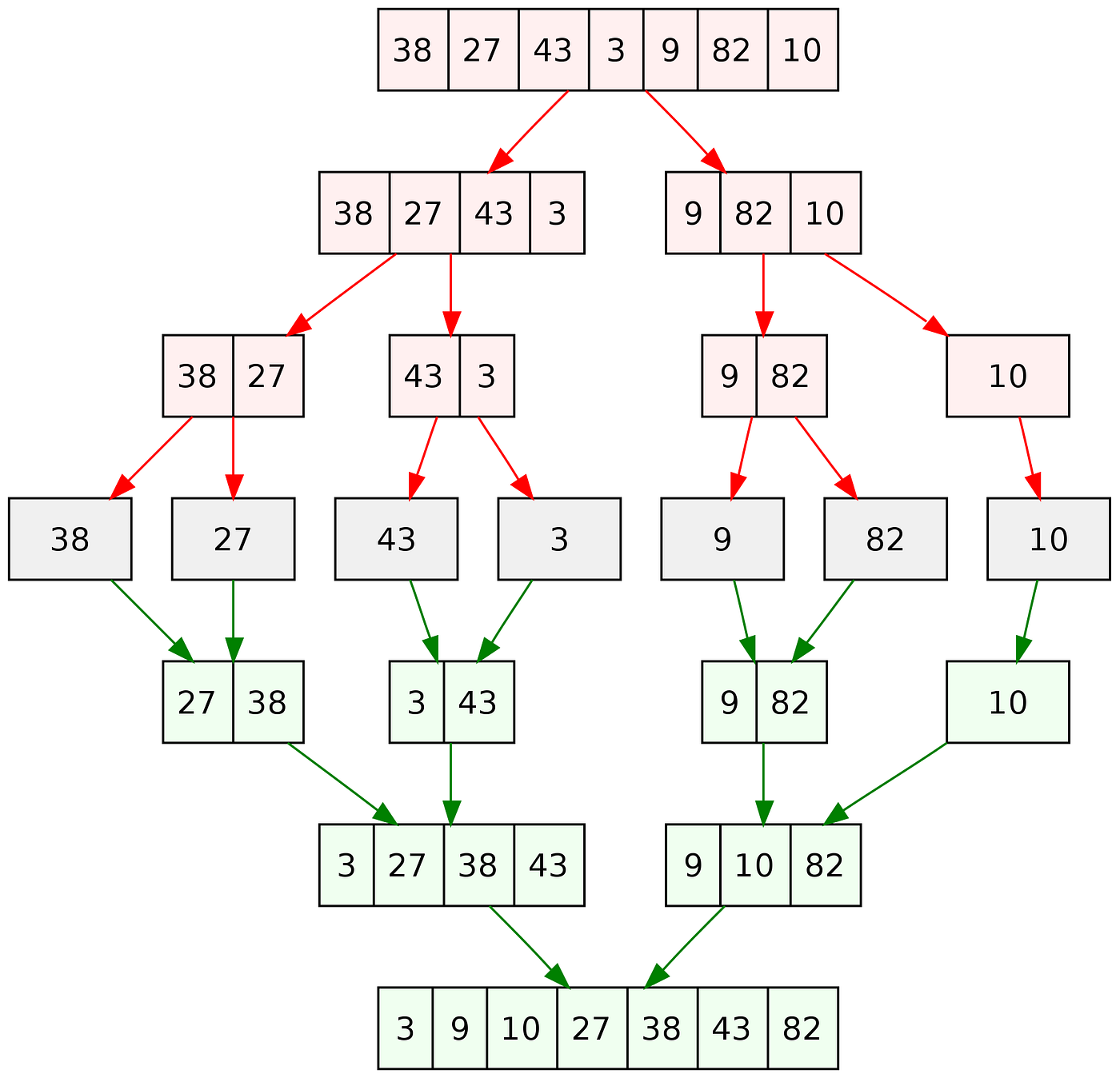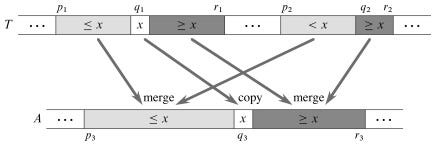# Concurrent Merge Sort in Golang

Back when I learnt merge sort, I always saw this diagram of dividing the array till the end and merging them in a sorted manner. A passing thought came to my mind, what if we could run the two halves in parallel like the diagram showed. Found out later like any other inventions, I am too late to the game. But felt content that my hunch was right and it has been attempted.Classical Merge sort [Photo by Vineet Kumar at English Wikipedia / Public domain]

# The algorithm

Let’s take a look into the pseudo-code and understand.

`A[p...r] : arrayp : start of arrayr : end of array MERGE_SORT(A, p, r)if p < r q = floor((p+r)/2) MERGE_SORT(A, p, q) // either this line  MERGE_SORT(A, q+1, r) // or this line can be run parallely MERGE(A, p, q, r) // a multi-threaded algorithm also exists for this function`

## Why didn’t we create two separate threads, for each call to the next level of merge_sort?

Threads can be light-weight but scheduling them can be more time-intensive than sequential execution. Besides, why have three instances of execution when we need two? It is better to have one function being executed at the parent process and another in a thread.

## How to make the merge function run concurrently?

Frankly speaking, I never thought we can have a non-serial (i.e. parallel) merge procedure. “Introduction to Algorithms”, (“CLRS” or “Cormen) book, has explained this in great detail.This is the diagram shamelessly copied from the book
• Here, x is the middle element, such that x = T[q1] where q1 is the middle index of the element.
• Since the subarray T[p1…r1] is sorted, all elements left of x are lesser than or equal to x.
• We then use binary sort to find the index q2 in T[p2…r2] such that the x can be inserted between T[q2–1] and T[q2] without any loss in sorting order.
• Before merging the newly divided subarrays, we place x between them in q3 position, which is determined by the length of the new left subarray
• We recursively merge
• T[p1…q1] with T[p2..q2]; new left sub array
• T[q1+1…r1] with T[q2…r2]; new right subarray
`T[] : original arrayT[p1...r1] : first sorted subarrayT[p2...r2] : second sorted subarrayA[p3...r3] : merged subarray outputP_MERGE(T, p1,  r1, p2, r2, A, p3) n1 = r1 - p1 + 1 // length of first subarray n2 = r2 - p2 + 1 // length of second subarray // Assuming n1 > n2 and both aubarrays are non-empty q1 = floor((p1+r1)/2) q2 = BINARY_SEARCH(T[q1], T, p2, r2) q3 = p3 + (q1-p1) + (q2-p2) A[q3] = T[q1] spawnn P_MERGE(T, p1, q1-1, p2, q2-1, A, p3) P_MERGE(T, q1+1, r1, q2, r2, A, q3+1) sync`

# The implementation

## Golang overview

Golang or Go is a relatively new language made in the age of multi-core processors. The designers of the language thus chalked out a very minimal yet powerful way to handle concurrency.

## Go routines

The main primitive used in Golang for concurrency is goroutine.

Just adding `go` keyword to a function makes that function a goroutine.

Goroutines are similar to application-level threads. Main differences are

• Goroutines are context switched on and off an OS thread instead of a OS core.
• Go schedulers are cooperative whereas OS schedulers are preemptive.
• i.e, context switches happen on purpose and not after elapsing a certain duration of time
• Go scheduling strategy is based on work-stealing

Work stealing : An under-utilised processor actively looks for other processor’s threads and “steal” some.

https://rakyll.org/scheduler/

## Wait Group

To wait for multiple go routines to finish, `WaitGroup` can be used.

# The code

## Tweak

There seems to be a tradeoff between the number of context switches between threads and actual execution of our code. So benchmark testing is usually done to determine a threshold to switch between sequential and parallel merge procedures based on the length of the array (slice, in Go terminology). Teiva Harsanyi has found the value to be around 1<<11.

# Conclusion

This article barely scratches the surface. Feel free to explore more and come up with suggestions.

If you liked my article, follow me on Medium and stay tuned for further updates.

I’m Srinjoy Santra, currently a SDE-1 at BookMyShow. You can connect with me on LinkedIn, visit my Github account, or follow me on Twitter.

(Originally published at https://thecodagram.com/merge-sort-in-golang/)

--

--